# Turboproofs, light clients and saving private Eth1

The Ethereum state is growing fast and left unchecked, chain management will only be accessible to a handful of big players. At his request, this article describes my interpretation of Alexey’s turboproof proof system, which is to be applied to several light client approaches.

The state of the Ethereum blockchain is stored as a hexary patricia tree, or trie for short. The data is stored at two levels:

1. The address trie is a mapping from addresses to account data.
2. A contract’s storage is also stored in a trie, which then represents a mapping from a 32-byte memory addresses to the 32–byte value stored at this address.

These trees store (key, value) pairs. Note that the keys’ base unit is the nibble (4 bits), not the byte.

The trees have 3 types of nodes:

• Leaves these are a (key suffix, value) pair, and they are always terminal nodes of the tree.
• Branch nodes these are internal nodes, and this node and all its subnodes share the same prefix. Each branch node has 17 entries. The first 16 entries correspond to the first nibble of the subnode’s key suffix. If present, the 17th entry is the value associated with the key prefix
• Extension nodes are “shortcut nodes” in case all the children share a common prefix. It is used to avoid creating a series of branch nodes, each with only one child.

As an example, the following tree has a collection of leaves, branch and extension nodes: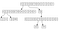Figure 1 A trie encoding the following values: (0x1234, 0x0000), (0x1111, 0x1111), (0x4567, 0x2222) and (0x4569, 0x3333). Keys and values have been shortened to 2 bytes in this example for increased readability. Rows with label 0 to 15 represent branch nodes, and arrows coming out of it specify what nibble its children have to be prefixed with. The 17th entry (index 16) is not used and therefore not represented. Row [5,6] is an extension node, meaning its children have to be prefixed with those two nibbles. Terminal nodes are leaves, the two on the left having key prefixes and the two on the right not needing a prefix as tracing the path down to them yields the whole key.

In practice, this model is the source of many efficiency issues in Ethereum, but it has also proven its resilience.

# Serializing values

Building on the previous example, here is the proof that both `(0x1234, 0x0000)` and `(0x4567, 0x2222)` are present in the tree: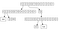Figure 2 A proof that the tree in figure 1 contains (0x1234, 0x0000) and (0x4567, 0x2222). All the subtrees leading to values other than these two are replaced with hashes (in this diagram, those subtrees are simple leaves).

Calculating the hash of the tree in figure 2 will yield the same root as the tree in figure 1, since the hashes provided in the proof have the same value as what would be provided if the tree was complete.

The question is how to serialize the data: given a list of hashes and a list of (key, value) pairs, how does one find the structure of the tree? For instance, given only the following input:

• (key, value) pairs (key and value lengths are shortened to make the resulting pictures more readable) for `(0x1234, 0x0000)` and `(0x4567, 0x2222)`
• Hashes representing the subtrees.

One could reconstruct the following tree: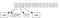Figure 3 An incorrect tree reconstruction for lack of structural information.

Or this tree: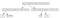Figure 4 Another, incorrect tree reconstruction for lack of structural information.

As a result, there is a need to somehow encode structural information.

# Turboproofs

A turboproof is broken into three parts:

1. The list of leaves
2. The list of hashes, corresponding to untouched branches of the tree
3. “Structural information”, that is, a list of instructions explaining how the tree can be rebuilt using only the hashes and leaves provided.

The last part is encoded as a list of instructions for a stack machine, to be executed to reconstruct the tree:

• `LEAF` indicates that a leaf should be popped from the proof’s sequence of leaves;
• `BRANCH(i)` stipulates that a new branch node needs to be created, and the node that was constructed before should be stored as the ith child of the new branch node. The new node is then stored on the stack;
• `ADD(i)` stipulates that given a branch node and any other node, the latter should be set as the ith child of the branch node. When one of the nodes isn’t a branch node, the order doesn’t matter. When both are branch nodes, the parent node will be the one that is on top of the stack;
• `EXTENSION(ext)` stipulates that the node of top of the stack should be set as the child of an extension node (a.k.a `shortNode` in geth parlance), whose prefix for the subtree is represented by the sequence of nibbles `ext`; and
• `HASH` is a node that represents the hash of a subtree

# Example

## The tree

(For clarity, keys are shortened to 4 bytes instead of the usual 32 bytes, and values are 8 bytes long, instead of having their size unrestricted)

The tree representation of these values is as follows:

## The proof

• The nodes are serialized in a depth-first order:
• The hashes are also serialized in a depth-first order. There’s only one hash, representing the `0xd*` subtree
• The set of instructions used to rebuild the tree:
`LEAFLEAFBRANCH(14)ADD(13)EXTENSION([0xa, 0xf, 0xe])BRANCH(13)HASHADD(14)`

The user now has the proof that they know the current state of the tree. They send it to the relayer or whoever wants to ensure that the user knows the part of the state they manage.

## Reconstructing the tree

• `LEAF`
• `LEAF`
• `BRANCH(14)`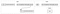Figure 10 The node at the top of the stack is popped, and set as the 14th child of a branch instruction. The sub-tree thus composed is immediately pushed back on top of the stack.
• `ADD(13)`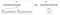Figure 11 The two elements at the top of the stack are popped, and the second one is added as the 13th child of the first one. The result is pushed back onto the stack.
• `EXTENSION([0xa, 0xf, 0xe])`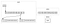Figure 12 The node at the top of the stack is popped and set as the child of an extension node with `0xafe` as prefix. The result is pushed back onto the stack.
• `BRANCH(13)`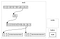Figure 13 The last node is taken from the proof’s node list and the subtree at the top of the stack is set as its 13th child. The result is, again, pushed to the stack.
• `HASH`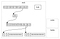Figure 14 A hash is taken from the proof’s list of hashes and pushed to the top of the stack.
• `ADD(14)`

The program has now terminated, and the top of the stack has the final version of the tree. This tree and the tree in figure 6 have the same root hash and it is trivial to verify that both keys are present.

# Relevance

With such proofs, it is possible for a user to only store the data they are interested in, and to prove ownership when they want to interact with the blockchain. This is known as a light client.

Pushing the concept further, one can envision a “stateless” future for the main chain (independently of what happens with Serenity), where users only keep the proof of their part of the state on the blockchain, and publish these information when they need to use it. This will help stop the inflation of the state and keep Ethereum accessible to all.

There’s still work to be done in order to keep the proof sizes small and quick to process, and hopefully making the use of these more common will help.

Written by